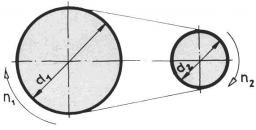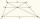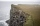# V-belt

Calculate a length of the V-belt when the diameter of the pulleys is:

D1 = 600 mm
D2 = 120 mm
d = 480 mm

Correct result:

l =  2185 mm

#### Solution:We would be pleased if you find an error in the word problem, spelling mistakes, or inaccuracies and send it to us. Thank you!Tips to related online calculators
Do you want to convert length units?

#### You need to know the following knowledge to solve this word math problem:

We encourage you to watch this tutorial video on this math problem:

## Next similar math problems:

• Trapezium diagonalsIt is given trapezium ABCD with bases | AB | = 12 cm, |CD| = 8 cm. Point S is the intersection of the diagonals for which |AS| is 6 cm long. Calculate the length of the full diagonal AC.
• Diagonals at right angleIn the trapezoid ABCD, this is given: AB=12cm CD=4cm And diagonals crossed under a right angle. What is the area of this trapezoid ABCD?
• Rectangular trapezoidThe ABCD rectangular trapezoid with the AB and CD bases is divided by the diagonal AC into two equilateral rectangular triangles. The length of the diagonal AC is 62cm. Calculate trapezium area in cm square and calculate how many differs perimeters of the
• Area of iso-trapFind the area of an isosceles trapezoid if the lengths of its bases are 16 cm and 30 cm, and the diagonals are perpendicular to each other.
• MO Z9–I–2 - 2017In the VODY trapezoid, VO is a longer base and the diagonal intersection K divides the VD line in a 3:2 ratio. The area of the KOV triangle is 13.5 cm2. Find the area of the entire trapezoid.
• Isosceles trapezoidIn an isosceles trapezoid KLMN intersection of the diagonals is marked by the letter S. Calculate the area of trapezoid if /KS/: /SM/ = 2:1 and a triangle KSN is 14 cm2.
• Trapezoid IVIn a trapezoid ABCD (AB||CD) is |AB| = 15cm |CD| = 7 cm, |AC| = 12 cm, AC is perpendicular to BC. What area has a trapezoid ABCD?
• See harmonicsIt is true that the size of the central segment of any trapezoid is the harmonic mean size of its bases? Prove it. Central segment crosses the intersection of the diagonals and is parallel to the bases.
• Trapezoid 25Trapezoid PART with AR||PT has (angle P=x) and (angle A=2x) . In addition, PA = AR = RT = s. Find the length of the median of Trapezoid PART in terms of s.
• Railway embankmentThe section of the railway embankment is an isosceles trapezoid, the sizes of the bases of which are in the ratio 5: 3. The arms have a length of 5 m and the height of the embankment is 4.8 m. Calculates the size of the embankment section area.
• Isosceles trapezoidFind the area of an isosceles trapezoid with bases of 8cm and 72mm. The height of the trapezoid is equal to three-quarters of the longer base.
• A cliffA line from the top of a cliff to the ground passes just over the top of a pole 5 ft high and meets the ground at a point 8 ft from the base of the pole. If the point is 93 ft from the base of the​ cliff, how high is the​ cliff?
• Vertical rodThe vertical one meter long rod casts a shadow 150 cm long. Calculate the height of a column whose shadow is 36 m long at the same time.The nine-meter poplar casts a shadow 16.2 m long. How long does a shadow cast by Peter at the same time, if it is 1.4 m high?One of the bases of the trapezoid is one-fifth larger than its height, the second base is 1 cm larger than its height. Find the dimensions of the trapezoid if its area is 115 cm2Calculate the height of the factory chimney, which casts a shadow 6.5 m long in the afternoon. At the same time, a 6 m high tree standing near it casts a shadow 25 dm long.Triangle A'B'C 'is similar to triangle ABC, whose sides are 5 cm, 8 cm, and 7 cm long. What is the length of the sides of the triangle A'B'C ' if its circumference is 80 cm?